Absolute Value
Exponents
Order of Operations
Graphing
Distributive Property
Percent
Slopes
100

Find the absolute value of:

|-10|100

When you see the following expression, what is the big number called and what is the little number on top of it called?

24

100

Simplify the following expression: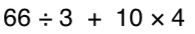100

Graph the following equation

y = 4x - 5

100

8(4+2) = ?

48

100

What is 25% of 80

20

100

what is the slope-intercept form equation?

y = mx + b

200

What is greater:

|-6| or |3|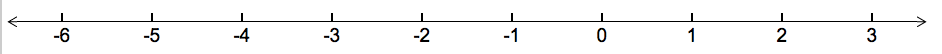The absolute value of a number is the number's distance from zero.

200

How can you rewrite 9in expanded form?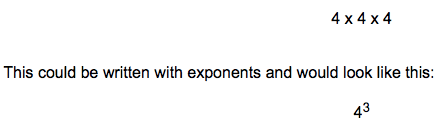200

Simplify the following expression: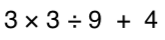200

y = (4/3)x -1

200

5(x-3)

5x-15

200

What is 15% of 120

18

200

what does b stand for in y=mx + b

y-intercept

300

Place the following in order from smallest number, to biggest number:

|-20|, 18, |12|, |-14|, 8

300

How can you rewrite the following in exponential form?

7 x 7 x 7 x 7 x 7300

3 + 42 * (3 + 2)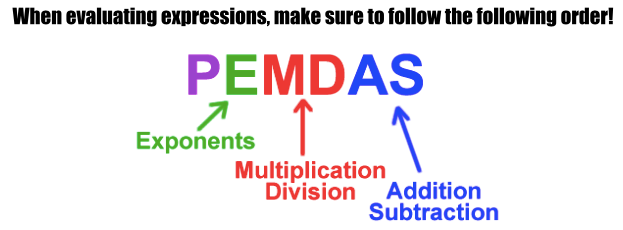300
y = -2x + 1
300

7+9(2x-3)

18x-20

300

130% of 42

54.6

300

what does m stand for in y=mx + b

slope (rise/run)

400

What is:

|-7| + |-2|

The absolute value of a number is the number's distance from zero.

400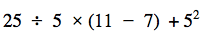400

y = 3

400

-3(-x-10)

3x+30

400

8% of 540

43.2

400

Turn the following standard form equation into a slope-intercept equation:

5x + 2y = 6

y = -5/2x + 6

500

2 x |-5| + |4| - 14

Remember to use your order of operations.

500500

x = -1

500

x + 10 -6(x-3x) +4

13x+14

500

70% of 478

334.6

500

Turn the following standard form equation into a slope-intercept equation:

-4x + 6y = -24

y=4/6x - 4

or y = 2/3x - 4

Click to zoom